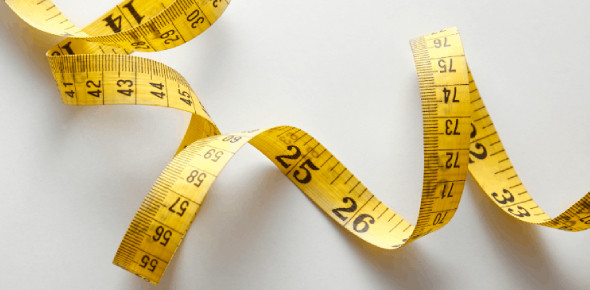# Quiz On Unit Of Measurement! Trivia Test

10 Questions | Attempts: 587
ShareSettingsAre you familiar with units of measurement? Unit of measure is a finite magnitude of a quantity, defined and developed by conversion or by law, employed as a standard for measurement of the same kind of capacity. Measurement is a method of determining how large or small a physical quantity is compared to a basic reference amount of the same type. If you are interested in learning more about units of measurement, complete this quiz.

• 1.
What would be the best unit to measure the capacity of a swimming pool?
• A.

Cup

• B.

Quart

• C.

Pint

• D.

Gallon

• 2.
You want to measure how much a dog weighs. Which unit of measurement would you use?
• A.

Inches

• B.

Pounds

• C.

Quarts

• D.

Yards

• 3.
What unit would you use to measure the height of a plant?
• A.

Inches

• B.

Pounds

• C.

Cups

• D.

Grams

• 4.
What unit would you use to measure the weight of an envelope?
• A.

Centimeter

• B.

Inch

• C.

Ounce

• D.

Pint

• 5.
What unit would you use to measure the capacity of a bathtub?
• A.

Yards

• B.

Gallons

• C.

Inches

• D.

Feet

• 6.
What unit would be the best for to measure the capacity of a fish tank?
• A.

Pints

• B.

Inches

• C.

Pounds

• D.

Grams

• 7.
What unit would you use to measure the length of a marker?
• A.

Grams

• B.

Kilograms

• C.

Centimeters

• D.

Kilometers

• 8.
What unit would you use to measure how much you weigh?
• A.

Kilometers

• B.

Centimeters

• C.

Liters

• D.

Pounds

• 9.
The length of Amanda's crayon is six _______ long.
• A.

Feet

• B.

Yards

• C.

Centimeters

• D.

Cups

• 10.
The weight of an envelope is two ______.
• A.

Feet

• B.

Ounces

• C.

Pints

• D.

Quarts

## Related TopicsBack to top
×

Wait!
Here's an interesting quiz for you.